Study Materials

# NCERT Solutions for Class 9th Mathematics

Page 5 of 9

## Chapter 13. Surface Areas and Volumes

### Exercise 13.5

EXERCISE 13.5

Q1. A matchbox measures 4 cm × 2.5 cm × 1.5 cm. What will be the volume of a packet containing 12 such boxes ?

Solution:

Length = 4 cm,  breadth = 2.5 cm,  height = 1.5 cm

Volume of cuboid = l × b × h

4 × 2.5 × 1.5 cm

10 × 1.5

15 cm × 3

180 cm3

Q2. A cuboidal water tank is 6 m long, 5 m wide and 4.5 m deep. How many litres of water can it hold? (1 m3 = 1000 l)

Solution:

Length = 6 m,  width = 5 m,  depth = 4.5 m

Volume of cuboid = l × b × h

6 × 5 × 4.5 m

30 × 4.5

135 m3

Water can be hold = 135 × 1000

135000 l

Q3. A cuboidal vessel is 10 m long and 8 m wide. How high must it be made to hold 380 cubic metres of a liquid?

Solution:

Length = 10 m,  width = 8 m,  volume = 380 cm2

Volume of cuboid = l × b × h

380 cm2 = 10 × 8 × h

380 cm2 = 80hQ4. Find the cost of digging a cuboidal pit 8 m long, 6 m broad and 3 m deep at the rate of 30 per m3.

Solution:

Length = 8m,  breadth = 6m,  height = 3m

Volume of cuboid = l × b × h

8 × 6 × 3 m

48 × 3 m

144 m3

Cost of digging = 144 × 30

Rs. 4320

Q5. The capacity of a cuboidal tank is 50000 litres of water. Find the breadth of the tank, if its length and depth are respectively 2.5 m and 10 m.

Solution:

1m3 = 1000 l

50000 l = 50 m3

Volume of cuboid = l × b × h

50m3 = 2.5 × 10 × h

50m3 = 25h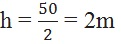Q6. A village, having a population of 4000, requires 150 litres of water per head per day. It has a tank measuring 20 m × 15 m × 6 m. For how many days will the water of this tank last?

Solution:

Population = 400

Per head per day = 150 l

Total water require per day = 400 × 150

600000 Litre

Volume of tank = 20 × 15 × 6 m

1800 m3  =   1800000 l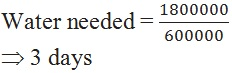Q7. A godown measures 40 m × 25 m × 15 m. Find the maximum number of wooden crates each measuring 1.5 m × 1.25 m × 0.5 m that can be stored in the godown.

Solution :

Volume of godown = l × b × h

40 × 25 × 15 m

15000 m3

Volume of crates = l × b × h

1.5 × 1.25 × 0.5 m

0.9375 m3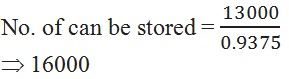Q8. A solid cube of side 12 cm is cut into eight cubes of equal volume. What will be the side of the new cube? Also, find the ratio between their surface areas.

Solution:

Volume of cube = a3

12 × 12 × 12

1728 cm3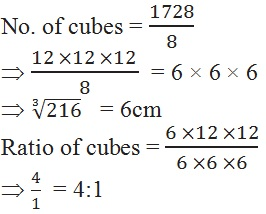Q9. A river 3 m deep and 40 m wide is flowing at the rate of 2 km per hour. How much water will fall into the sea in a minute?

Solution:

Deep = 3m,  width = 40 m,  speed = 2 kmph

Volume of river = 2000 × 40 × 3

24000 m3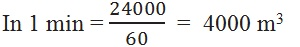Page 5 of 9

Chapter Contents: